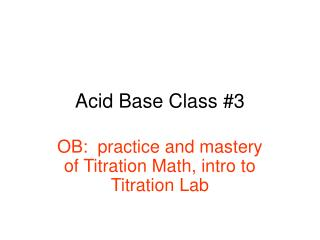DownloadDownload PresentationAcid Base Class #3

# Acid Base Class #3

Télécharger la présentation## Acid Base Class #3

- - - - - - - - - - - - - - - - - - - - - - - - - - - E N D - - - - - - - - - - - - - - - - - - - - - - - - - - -
##### Presentation Transcript

1. Acid Base Class #3 OB: practice and mastery of Titration Math, intro to Titration Lab

2. Arrhenius Acid + Arrhenius Base Salt + water HCl(AQ) + NaOH(AQ) NaCl(AQ) + HOH(L) In an acid base neutralization that is balanced, each hydrogen ion is combined with a hydroxide ion. Each pair makes a molecule of water. If it is truly neutralized, there are no left over ions, they are all balanced into water. In most cases, without careful measuring, and knowledge of the strengths of the acid and base, you could not easily neutralize an acid and base. There is math to help us. It’s called TITRATION MATH, because it’s used with the lab technique called titration, where neutralization occurs.

3. We’ll use a burette to measure acids and bases carefully. They’re long glass tubes with lots of lines to measure the solutions. They have very fine nozzles, that will allow only the smallest drops out at a time (or a thin rushing stream). Base of measured volume, known molarity Also contains an ACID BASE indicator Acid in beaker, known volume + UNKNOWN MOLARITY

4. By dripping in slowly the base, at some point the indicator will change colors, telling us we are at neutral. We can measure how much base we used, and we measured the volume of acid also. Time for MATH. Base of measured volume, known molarity Also contains an ACID BASE indicator Acid in beaker, known volume + UNKNOWN MOLARITY

5. (Molarity of Acid)(volume of acid) = (molarity of Base)(volume of base) (MA)(VA) = (MB)(VB) Question #1… How much NaOH base of 0.250M is needed to neutralize 83.0 mL of 1.00 M HCl acid?

6. (MA)(VA) = (MB)(VB) Question #1… How much NaOH base of 0.250M is needed to neutralize 83.0 mL of 1.00 M HCl acid? (MA)(VA) = (MB)(VB) (1.00M)(83.0 mL) = (0.250M)(VB) 332 mL = VB 3SF

7. Start Volume in mL End Volume in mL We measure “upside down” with burettes. Be careful. Measure to the nearest 10 mL.

8. We will use indicators to tell us when we reach neutral. Strangely we’ll use phenolphthalein, which changes from pH 8 to pH 9. How can this be, if we’re looking for neutral??? It will get slightly complicated right now. Neutral is when the # the H+1 ions = # OH-1

9. At pH 7.000, the concentration of H+1 = concentration of OH-1 ions. This can be written as [H+1] = [OH-1 ] In lab, when your acid and base are being neutralized in your mixing beaker, you are within one drop of neutral when you use phenolphthalein. You can’t get much closer than that in high school chem lab. You could use an electronic pH meter to get to exactly 7.0000 pH, but it’s really close enough anyway. Besides, we all know you won’t read the burette scale perfectly anyway.It’s just percent error.

10. Next problem.. Your burette has 48.0 mL of 1.20 M NaOH and you titrate 75.0 mL HCl that has a few drops of phenolphthalein indicator. You end with 27.0 mL of NaOH in the buret, and you are as close to neutral as you can measure. What is the molarity of the acid? (hint, first figure out how much of the base you used)

11. Your burette has 48.0 mL of 1.20 M NaOH and you titrate 75.0 mL HCl that has a few drops of phenolphthalein indicator. You end with 27.0 mL of NaOH in the buret, and you are as close to neutral as you can measure. What is the molarity of the acid? (MA)(VA) = (MB)(VB) (MA)(75.0 mL) =(1.20M)(21.0 mL) MA = 0.336 M

12. It takes 12.4 mL of 1.90 M HCl to exactly neutralize 104 mL of NaOH. What is the molarity of the base?

13. 13

14. It takes 12.4 mL of 1.90 M HCl to exactly neutralize 104 mL of NaOH. What is the molarity of the base? (MA)(VA) = (MB)(VB) (1.90 M)(12.4 mL) = (MB)(104 mL) 0.227 M = MB

15. You need 782.2 mL of base to neutralize exactly 1500. mL nitric acid that has a 4.56 M. What is the molarity of this base? Hint: write the formula every time!

16. You need 782.2 mL of base to neutralize exactly 1500. mL nitric acid that has a 4.56 M. What is the molarity of this base? (MA)(VA) = (MB)(VB) (4.56 M)(1500. mL) = (MB)(782.2 mL) 8.74 M = MB

17. NOTE: Please go back over your notes today and see that each of the acids and each of the bases are SINGLE ion compounds. Every acid today has just one H+1 per molecule. Each base today has just one OH-1 per molecule. That makes balancing the ions to neutral very easy. Tomorrow we’ll glance at neutralizations like H2SO4 + KOH (this is a 2:1 ion ratio), or, H3PO4 + Ca(OH)2 (which is 3:1 ion ratio) Due Immediately: Solutions Lab, Clock Lab Due Fri: Acid Base HW #2 – Titration Math practice